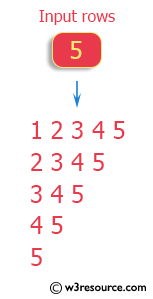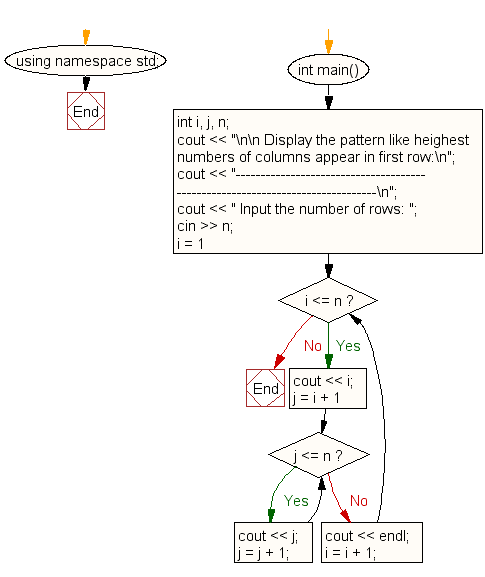﻿ C++ : Rows with highest number of columns appear first

# C++ Exercises: Print a pattern like highest numbers of columns appear in first row

## C++ For Loop: Exercise-50 with Solution

Write a C++ program to print a pattern in which the highest number of columns appears in the first row.

Pictorial Presentation:Sample Solution:

C++ Code :

``````#include <iostream>
using namespace std;

int main()
{
int i, j, n;
cout << "\n\n Display the pattern like highest numbers of columns appear in first row:\n";
cout << "------------------------------------------------------------------------------\n";
cout << " Input the number of rows: ";
cin >> n;
for (i = 1; i <= n;)
{
cout << i;
for (j = i + 1; j <= n;)
{
cout << j;
j = j + 1;
}
cout << endl;
i = i + 1;
}
}
``````

Sample Output:

``` Display the pattern like highest numbers of columns appear in first row:
------------------------------------------------------------------------------
Input the number of rows: 5
12345
2345
345
45
5
```

Flowchart:C++ Code Editor: